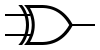## Problem A: XORGiven two integers S and F, what is the XOR (exclusive-or) of all numbers between S and F (inclusive)?

### Input Specification

The first line of input is the integer T, which is the number of test cases (1 ≤ T ≤ 1000).

T lines follow, with each line containing two integers S and F (1 ≤ SF ≤ 1,000,000,000).

### Output Specification

For each test case, output the (decimal) value of the XOR of all numbers between S and F, inclusive.

```5
3 10
5 5
13 42
666 1337
1234567 89101112
```

### Sample Output

```8
5
39
0
89998783
```

Point Value: 7
Time Limit: 2.00s
Memory Limit: 16M